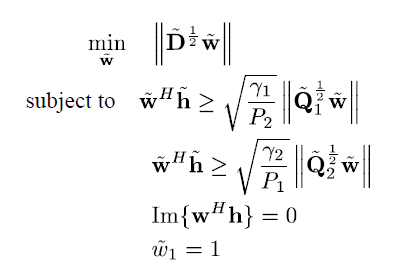# Issues with a convex SOCP problem

Hello,

I am trying to solve the following convex SOCP problem with CVX. My problem is that CVX-status shows “Solved” state but when I check the constraints, they are not satisfied. I was wondering if you can help me to find my mistake here?

%—values--------------------------------------------
gamma = .1;
R = 6;
Lw = 5;
P1 = 41.14;
P2 = 19.79;

D = P1D1+P2D2+eye(RLw);
h_tild = [0;h];
D_tildq = ([0,zeros(1,R
Lw);zeros(RLw,1), D])^(.5);
Q1_tildq = ([1,zeros(1,R
Lw);zeros(RLw,1), P2QI+D1])^(.5);
Q2_tildq = ([1,zeros(1,RLw);zeros(RLw,1), P1*QI+D2])^(.5);
a1 = sqrt(gamma/P2);
a2=sqrt(gamma/P1);

%—optimization----------------------------------------------------
cvx_begin quiet
cvx_precision best
cvx_solver Mosek
variable w(RLw+1) complex
minimize( norm(D_tildq
w) )

``````subject to
w(1)==1;
imag(w(2:end)'*h)==0;   %(w'*h_tild)
real(w'*h_tild) >=  a1*norm(Q1_tildq*w);
real(w'*h_tild) >=  a2*norm(Q2_tildq*w);
``````

cvx_end

cvx_status

%—check the constraints---------------------------
const1=real( (w’h_tild) - a1(norm(Q1_tildqw)) )
const2=real( (w’h_tild) - a2(norm(Q1_tildq
w)) )

Here is the link to the matrices that I am using for this run and also the m-file for this example:

Here is the problem formulation:Any help would be appreciated!

What is cvx_status reported as? If the problem has been reported as solved, then

How big are the constraint violations? Solvers allow themselves a tolerance for producing a solution which is not exactly feasible.

Cvx_status is “solved”. But instead of getting a positive constraint, the constraint is a very small negative value, for example in the order of -1e-9.
Would you please explain what do you mean by the solver tolerance?
Does it mean the solution is not reliable?

That is within solver tolerance of feasibility. If you don’t like that, either adjust the solver feasibility tolerance using `cvx_solver_settings` http://cvxr.com/cvx/doc/solver.html#advanced-solver-settings (but you can’t make solver feasibility tolerance too small, given use of double precision) or add 1e-6 or 1e-7 (or maybe 2e-8) to the right hand side of the inequality to ensure that the solver solution will be strictly feasible if you really require that.